# NCERT Solutions for Class 9 Maths Chapter 6 Lines And Angles

NCERT solutions for class 9 maths chapter 6 Lines And Angles- When two points are joined together, the resultant is called line. An angle is formed when two unparalleled lines are connecting at a point. These two lines are called the sides of the angle. In the previous chapter, you have already studied some basic formations. In this chapter, we will learn concepts of vertically opposite angles, supplementary angles, and complementary angles and also study properties of parallel lines and transversal lines, intersecting lines, non-intersecting Lines and angle sum property of the triangle. Solutions of NCERT for class 9 maths chapter 6 Lines and Angles covers the solutions from every concept. This chapter contains 3 exercises with a total of 18 questions. CBSE NCERT solutions for class 9 maths chapter 6 Lines And Angles carry a detailed explanation to all the 18 questions in practice exercises. Lines and angles are everywhere around us. To design the structure of the building, an architecture uses both lines and angles. When we have to find the height of a building or a tower, then we need to know angles. NCERT solutions for class 9 maths chapter 6 Lines and Angles will also be helpful in the preparation NTSE, Indian National Olympiad (INO), etc. Finally, it can be concluded that the NCERT solutions can play a vital role in increasing your scores.

## Some basic terms and their figures that we are going to discuss in NCERT Class 9 Maths Chapter Lines And Angles are

 Terms Figures Acute angleRight angleObtuse angleStraight angleReflex angle## Q1 In Fig. 6.13, lines AB and CD intersect at O. If AOC + BOE = 70° and BOD = 40°, find BOE and reflex COE.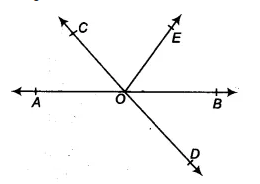Given that,
AB is a straight line. Lines AB and CD intersect at O.  and  BOD =
Since AB is a straight line
AOC + COE + EOB =
[since ]

So, reflex COE =
It is given that AB and CD intersect at O
Therefore, AOC  = BOD  [vertically opposite angle]
[ GIven  BOD = ]
Also,
So,  BOE =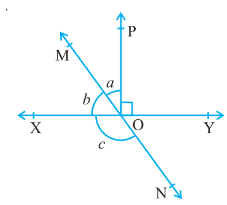Given that,
Line XY and MN intersect at O and POY =  also ..............(i)

Since XY is a straight line
Therefore, ...........(ii)
Thus, from eq (i) and eq (ii), we get

So,
Since MOY = c [vertically opposite angles]
a + POY = c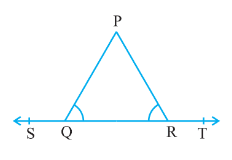Given that,
ABC is a triangle such that  PQR =  PRQ  and ST is a straight line.
Now,  PQR +  PQS =      {Linear pair}............(i)
Similarly,  PRQ +  PRT = ..................(ii)

equating the eq (i) and eq (ii), we get

{but  PQR =  PRQ }
Therefore,  PQS = PRT
Hence proved.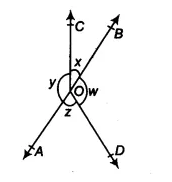Given that,
..............(i)
It is known that, the sum of all the angles at a point =
..............(ii)

From eq (i) and eq (ii), we get

Hence proved AOB is a line.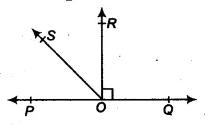Given that,
POQ is a line, OR  PQ and  ROQ is a right angle.
Now,  POS + ROS +  ROQ =   [since POQ is a straight line]
.............(i)
and,  ROS +  ROQ =  QOS
..............(ii)

Add the eq (i ) and eq (ii),  we get

hence proved.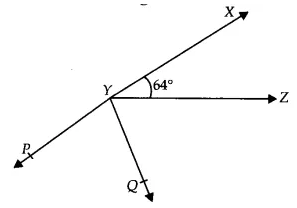Given that,
XYZ =  and XY produced to point P and Ray YQ bisects ZYP
Now, XYP is a straight line
So, XYZ + ZYQ + QYP =

Thus reflex of QYP =

Since XYQ = XYZ + ZYQ  [

## NCERT solutions for class 9 maths chapter 6 Lines and Angles Excercise: 6.2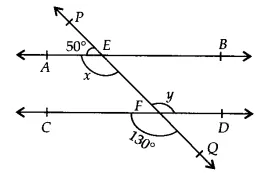Given that,
In the figure, CD and PQ intersect at  F
Therefore,   (vertically opposite angles)

PQ is a straight line. So,

Hence AB || CD (since  and are  alternate interior angles)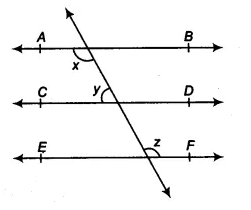Given AB || CD and CD || EF and
therefore, AB || EF and  (alternate interior angles)..............(i)

Again, CD || AB
.............(ii)

Put the value of  in equation (ii), we get

Then

By equation (i), we get the value of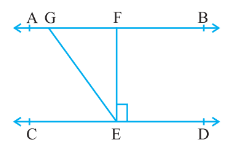Given AB || CD, EFCD and GED =
In the above figure,
GE is transversal. So, that AGE = GED =   [Alternate interior angles]
Also, GEF = GED - FED
=

Since AB is a straight line
Therefore, AGE  + FGE =
So, FGE =Draw a line EF parallel to the ST through R.
Since PQ || ST and ST || EF
EF || PQ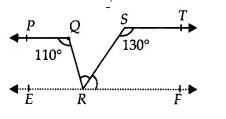PQR = QRF =   (Alternate interior angles)
QRF = QRS + SRF .............(i)

Again, RST + SRF =  (Interior angles of two parallels ST and RF)
(RST = , given)

Thus, QRS =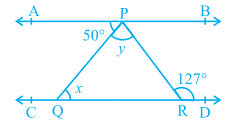Given, AB || CD, APQ =  and PRD =
PQ is a transversal. So,
APQ = PQR=  (alternate interior angles)

Again, PR is a transversal. So,
y + =  (Alternate interior angles)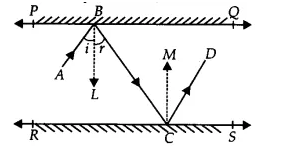Draw  a ray BL PQ and CM  RS
Since PQ || RS (Given)
So, BL || CM and BC is a transversal
LBC =  MCB (Alternate interior angles).............(i)

It is known that, angle of incidence  = angle of reflection
So, ABL = LBC and MCB =  MCD
..................(ii)

Adding eq (i) and eq (ii), we get

ABC = DCB
Both the interior angles are equal
Hence AB || CD

## NCERT solutions for class 9 maths chapter 6 Lines and Angles Excercise: 6.3

### Q1 In Fig. 6.39, sides QP and RQ of PQR are produced to points S and T respectively. If  SPR = 135° and PQT = 110°, find PRQ.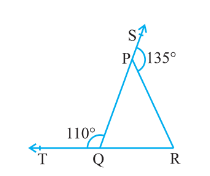Given,
PQR is a triangle, SPR =,  PQT =

Now, TQP + PQR =  (Linear pair)
So, PQR =

Since the side of QP of the triangle, PQR is produced to S
So, PQR + PRQ =  (Exterior angle property of triangle)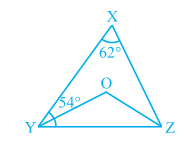We have
X = XYZ =
YO and ZO bisects the XYZ and XZY
Now, In XYZ, by using angle sum property
XYZ + YZX + ZXY =

So, YZX =
YZX =

and, OYZ =  also, OZY =

Now, in OYZ
Y + O + Z =   [Y =  and Z = ]
So, YOZ =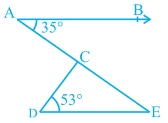We have,
AB || DE,  BAC = 35° and  CDE = 53°

AE is a transversal so,  BAC =  AED =

Now, In  CDE,
CDE + DEC + ECD =  (By angle sum property)
Therefore, ECD =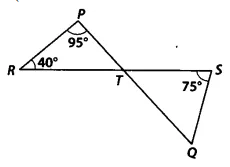We have,
lines PQ and RS intersect at point T, such that PRT = 40°, RPT = 95° and TSQ = 75°

In PRT, by using angle sum property
PRT + PTR + TPR =
So, PTR  =

Since lines, PQ and RS intersect at point T
therefore, PTR = QTS (Vertically opposite angles)
QTS =

Now, in QTS,
By using angle sum property
TSQ + STQ + SQT =
So, SQT =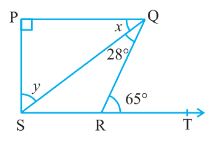We have,
PQ PS, PQ || SR, SQR = 28° and QRT = 65°
Now, In  QRS, the side SR produced to T and PQ || RS
therefore, QRT =  =
So,

Also, QRT = RSQ + SQR (By exterior angle property of a triangle)
Therefore, RSQ = QRT - SQR

Now, in  PQS,
P + PQS + PSQ =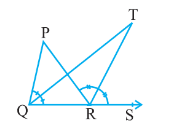We have,
PQR is produced to a point S and  bisectors of PQR and PRS meet at point T,
By exterior angle sum property,
PRS = P + PQR
Now,
................(i)

Since QT and QR are the bisectors of  PQR and PRS respectively.

Now, in QRT,
..............(ii)

From eq (i) and eq (ii),  we get

Hence proved.

## NCERT solutions for class 9 maths chapter wise

 Chapter No. Chapter Name Chapter 1 NCERT solutions for class 9 maths chapter 1 Number Systems Chapter 2 CBSE NCERT solutions for class 9 maths chapter 2 Polynomials Chapter 3 Solutions of NCERT class 9 maths chapter 3 Coordinate Geometry Chapter 4 NCERT solutions for class 9 maths chapter 4 Linear Equations In Two Variables Chapter 5 CBSE NCERT solutions for class 9 maths chapter 5 Introduction to Euclid's Geometry Chapter 6 NCERT solutions for class 9 maths chapter 6 Lines And Angles Chapter 7 NCERT solutions for class 9 maths chapter 7 Triangles Chapter 8 CBSE NCERT solutions for class 9 maths chapter 8 Quadrilaterals Chapter 9 Solutions of NCERT class 9 maths chapter 9 Areas of Parallelograms and Triangles Chapter 10 NCERT solutions for class 9 maths chapter 10 Circles Chapter 11 CBSE NCERT solutions for class 9 maths chapter 11 Constructions Chapter 12 Solutions of NCERT class 9 maths chapter 12 Heron’s Formula Chapter 13 NCERT solutions for class 9 maths chapter 13 Surface Area and Volumes Chapter 14 CBSE NCERT solutions for class 9 maths chapter 14 Statistics Chapter 15 Solutions of NCERT class 9 maths chapter 15 Probability

## How to use NCERT solutions for class 9 maths chapter 6 Lines and Angles

• Before coming to this chapter, please ensure that you know the concepts of the previous chapter.

• First, go through some basic terminologies and observations given in the NCERT textbook.

• learn the usage of these concepts in the questions.

• Use these concepts while solving the practice exercises and for the help, you can use NCERT solutions for class 9 maths chapter 6 Lines and Angles.

Keep Working Hard and Happy Learning!